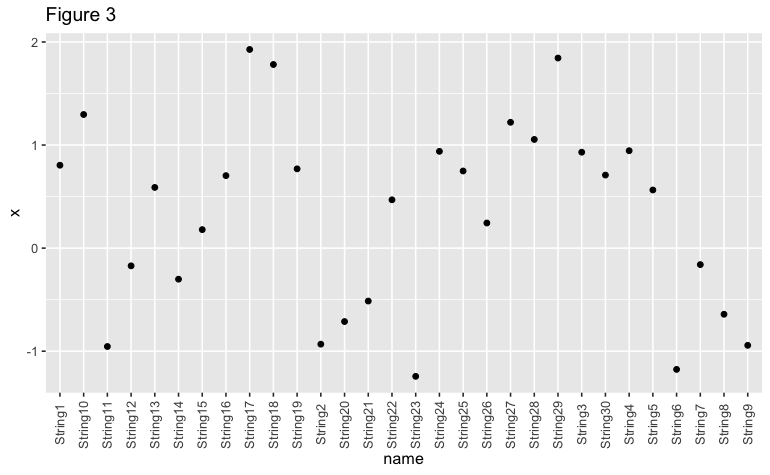# Avoid Overlapping Axis Labels in R (2 Examples)

This tutorial shows how to avoid overlapping axis labels in the R programming language.

The table of content is structured as follows:

Let’s take a look!

## Data Sample, Add On Packages & Default Plot

For this tutorial, we use the following data:

```set.seed(999)
data_sample <- data.frame(x = rnorm(30),
name = paste0("String",1:30))If not installed yet, please install the ggplot2 package in order to draw our sample data:

```install.packages("ggplot2")
library("ggplot2")```

If you have already installed ggplot2, you just need to load the package:

`library("ggplot2")`

Now, we can draw our data:

```ggplot(data_sample) +
geom_point(aes(name,x)) +
ggtitle("Figure 1")```As we can see in Figure 1, it’s almost impossible to read names in the x-axis due to the overlapping labels. What can we do about it?

## Example 1: Avoid Overlapping Axis Labels in a Graph Using guide_axis() Function

Fortunately, the latest version of ggplot2 (3.3.0) offers a quick solution to this problem. We can use a function called guide_axis() to avoid overlapping axis labels by stacking the labels:

```ggplot(data_sample) +
geom_point(aes(name,x)) +
scale_x_discrete(guide=guide_axis(n.dodge=3)) +
ggtitle("Figure 2")```The argument we are using inside guide_axis, “n.dodge=3”, arranges every three axis labels separately from the x-axis. We can change this argument depending on the length of the label names we are using in our data frame.

## Example 2: Avoid Overlapping Axis Labels in a Graph Using theme() Function

Another solution to avoid overlap in axis labels is to rotate the x-axis by using the theme() function that the ggplot2 package offers. In this case, we will rotate the x-axis labels by 90 degrees, but this rotation number can be changed as needed:

```ggplot(data_sample) +
geom_point(aes(name,x)) +
theme(axis.text.x = element_text(angle = 90,
vjust = 0.5,
hjust = 0.5)) +
ggtitle("Figure 3")```As shown in Figures 2 and 3, it is possible to create a ggplot2 plot that avoids an overlap in axis labels in R.

## Video, Further Resources & Summary

Do you need more explanations on how to avoid an overlap of axis labels? Then you should have a look at the following YouTube video of the Statistics Globe YouTube channel.

Furthermore, you could have a look at some of the other tutorials on Statistics Globe:

This post shows how to remove overlap for axis labels in R. In case you have further questions, you may leave a comment below.